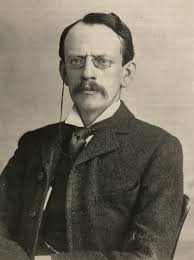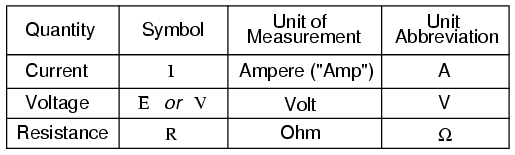# Ohm’s Law

## Ohm’s LawOhm’s Law

`

Many scientists’ relentless efforts resulted in electricity invention. German physicist, George Ohm was an outstanding contributor in this. He discovered a law of electronic conductivity between two points. He published his treatise on his research in 1827. Ohm uses simple electronic circuits with varied lengths of wires. He then describes voltage and current ‘flow’ in the wires and described how to measure them. This law by Ohm is known as Ohm’s law today.

It is,

I = V*R

or, V = I/R

Here,

I = Current

V = Voltage

R = Resistance

If we want to understand Ohm’s law better, we need to understand some basic factors/ideas related to electricity. Electricity is the movement of electrons. That means when electrons try to move from one place to another, it produces electricity.

We have to go back to the time of the scientists to see how Ohm found his law for electricity. Many scientists before Ohm started research on it.

At first, Henry Cavendish made an experiment in 1781. He used salt solution filled Leyden jars and glass tubes of different sizes and shapes. He used his body to complete the circuit and felt shock. He could not measure the shock for lack of preparation. But he suggested that varied “degree of electrification” (voltage) causes varied range of “velocity” (Current). His works were later published by Maxwell in 1879.

In 1814, Francis Ronalds used a gold-leaf electrometer to delineate Dry piles’ intensity (voltage) and quantity (current).

Joseph Fourier’s “The Analytic Theory of Heat” helped Ohm to a great extent. Ohm used his theories to explain his works theoretically. For finding out internal resistance and constant voltage, he used ‘voltaic piles’ at first. Later, ‘thermocouple’ was used by him as a more stable voltage source. Galvanometer measures current in his experiment. He found that the temperature and voltage between two thermocouple terminals were proportional. Wires of different length, diameter, and material were used to complete the circuit. All the data in his experiments can model an equation, the Ohm’s law.

Voltage, current, and resistance are the three basics of electricity. As these concepts are unseen, it is a little bit difficult to understand. Even Ohm used a complex process to determine them. But the processes have become easier for better understanding. New processes not only give basic idea on voltage, current, and resistance but also depict how they relate to each other.

Ohm was criticized for his discovery in his time. As electron was not yet discovered, what creates the shock was still unknown. Critics of his time called his work a "web of naked fancies". They believed that experiments should not defy natural order. The Educational minister at that time claimed that a professor with such fancies should not continue teaching profession anymore. His brother was a mathematician who fought against German education system for his recognition. His works remained unacceptable till 1940s. But he got due respect for his discovery before his death.

J. J. Thompson discovered the electron in 1897. This discovery clarified that it was electron is the particle to carry electric currents in electric circuits. Paal Drude proposed ‘Drude model’, the first classical model of electrical conduction. It finally gave the scientific explanation of Ohm’s law.We have got electrons. Let's now understand the basics. Atoms consist of electrons, protons, and neutrons. Protons and neutrons stay in the centre as the nucleus while electrons encircle the nucleus in a specific orbital. Every atom is electrically neutral as the number of electrons and protons are the same. If we add or remove electrons from a substance’s atoms, it becomes electrically positive or negative. Whether they be, they cause electricity flow.

Ohm’s law gives us an idea about ‘resistance’ that relates current and voltage. Voltage is the difference between two points in a circuit. Charges move from a higher point to a lower point creating a flow. Yes! Like water flow from a tank.

Think of a tank full of water. Here,

The water amount = charge

The water pressure = voltage

The water flow = current

If water flows through a pipe, the pressure can be named as voltage as the water in the tank is charge. The more amount of water, the more pressure. Same thing happens when extra charge creates extra voltage.

Now to understand ‘resistance’, we can take two tanks full of the same amount of water. But the pipes are different in size; one pipe is narrower than the other. So, the flow rate will definitely fluctuate. The narrower pipe will allow less water to flow. If want to have the same pressure from both narrow and wide pipe, we have to increase the water level in the narrow pipe containing tank.

It shows that, the narrow pipe resist water flow. So, we can say

Pipes width = Resistance

Two circuits with same voltage can be joined with wires of different resistances. The higher the resistance, the less charge flows through it.

The symbol, unit of measurement and unit abbreviation of the three are-Coming back to Ohm’s law, we can have a triangle or pyramid. As it relates to three electrical terms, we can find out any of them provided that two are given. The triangle is-We can find out current, voltage and resistance by using Ohm’s law. For example,

If the resistance between two points is 250 Ω and 0.2 A flowing through it, what is the voltage of it?

According to Ohm’s law

V = I*R

or, V = 0.2*250

or, V = 50 V

If the voltage between two points is 160V and 3.2A current flows through the resistance, what is the resistance of the electric iron?

Solution:

V = I*R

or, R = V/I

or, R = 160/3.2

or, R = 50Ω

Suppose, a voltage of 10 volts is placed across a wire that’s resistance is 50Ω. What is the current flow?

Solution:

V=I*R

or, I = V/R

or, I = 10/50

or, I = 0.02A

Ohm’s law has some applications. First of all, it determines voltage, resistance or current of an electric circuit. It helps to maintain the proper voltage drop in all electronic components in circuit. This law is also used in dc systems to divert the current.

Resistors are the immediate result of Ohm’s law. It added a new dimension in creating better electric devices. It increases the capacities of transistors. Circuits that work in direct current (DC) follows Ohm’s law. Alternative current (AC) also follows this rule until the resistor does not contain inductance or capacitance.

Most common types of resistors are carbon-composition resistors. Manufacturers use a mixture of graphite and clay to harden them. These resistors are made by maintaining a ratio between carbon and clay. The more the carbon’s rate, the more resistance it provides. Another form of resistor is ‘wirewound resistors. They are similar to carbon resistors in size, but they can handle more currents than them.

There are color bands calculator is a useful tool to decode information for color banded axial lead resistors. The number of color bands is very important as the change of the decoding depends on the number of color bands. four bands, five bands, and six bands resistors are the most common types of resistors. For four bands transistors, 1st band works for first significant digit, 2nd band for second significant digit, 3rd one for multiplier and the 4th one is for tolerance.

Although Ohm’s law works on both AC and DC current, it has some limitations too. Diodes and transistors are unilateral electrical elements. They allow only one direction current flow and that is why Ohm’s law does not apply here. Non-linear electric elements also make Ohm’s law not applicable because of some parameters.

We have seen Ohm’s law’s triangle covering three things. But, when ‘Power’ is added to the three it makes new laws. All the laws made by these four can be combined in Ohm’s law pie chart.Ohm’s law matrix table is also another smart table combining all the laws accordingly in a single table.